Latest Banking jobs   »

# Quantitative Aptitude Quiz For SIDBI Grade A Prelims 2023- 10th January

Directions (1-5): Each of the following questions below consists of a question and two statements numbered I and II given. You have to decide whether the data provided in the statements is sufficient to answer the questions.
(a) if the data given in statement, I alone is sufficient to answer the question while the data in statement II alone is not sufficient to answer the question.
(b) if the data given in statement II alone is sufficient to answer the question while the data in statement, I alone is not sufficient to answer the question.
(c) if the data either in statement I alone or in statement II alone is sufficient to answer the question.
(d) If the data in neither statement I nor II is sufficient to answer the question.
(e) If the data in both statements I and II together is necessary to answer the question.

Q1. Find the cost price of article by shopkeeper on selling the article at Rs. 240.
(I) If the article sold at 25% more the profit earned will be Rs. 40.
(II) Marked price of article is Rs. 400 and profit% is equal to discount% and profit% is 40%.

Q2. Find the volume of right circular cone.
(I) Height of cone is 100% more than radius of cone.
(II) Area of base of cone is 154 cm².

Q3. Find the value of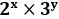.
(I) Sum of value of x and y is 8.
(II) Product of value of x & y is 7.

Q4. Find the speed of boat in still water.
(I) Time taken by boat to cover 64 km in downstream is half the time taken by same boat to cover same distance in still water.
(II) Speed of stream is 5 km/hr

Q5. In a box three types of balls are there, Black, Red and White. If no. of white balls is given then find out the probability of getting one white ball.
(I) Probability of getting one red ball is given.
(II) Probability of getting one black ball is given.

Direction (6 – 10): In the given questions, two quantities are given, one as ‘Quantity I’ and another as ‘Quantity II’. You have to determine the relationship between two quantities and choose the appropriate option:

Q6. – In how many days work will be completed if all the boys work together.
Quantity I- Ram can complete a work in 15days, Suraj can complete the work in 10days and Samir takes 20days to complete the same work alone.
Quantity II – Rohan, Ravi and Sanjeev take 16days, 24days and 8days to complete a certain work respectively.
(a) Quantity I > Quantity II
(b) Quantity I < Quantity II
(c) Quantity I ≥ Quantity II
(d) Quantity I ≤ Quantity II
(e) Quantity I = Quantity II or no relation

Q7. What will be the age of Suman.
Quantity I – The ratio between Suman’s and Raju’s present age is 9:11 and, Raju is 6 years elder to Suman.
Quantity II – 25years
(a) Quantity I > Quantity II
(b) Quantity I < Quantity II
(c) Quantity I ≥ Quantity II
(d) Quantity I ≤ Quantity II
(e) Quantity I = Quantity II or no relation

Q8. What is the S.I accrued on a principle of Rs.6000 in 5 years.
Quantity I – The rate of interest for the first 2 years is 10% and for the last 3 years is 15%.
Quantity II – Rs.3900
(a) Quantity I > Quantity II
(b) Quantity I < Quantity II
(c) Quantity I ≥ Quantity II
(d) Quantity I ≤ Quantity II
(e) Quantity I = Quantity II or no relation

Q9. What is the Cost Price of the fan.
Quantity I – If the shopkeeper sells a fan at Rs1600 with 20% loss
Quantity II – If the marked price of an item is 60% more than CP, and if he gives two successive discounts of 10% and 20% respectively, then his overall profit Rs. 304. Find cost price of the item.
(a) Quantity I > Quantity II
(b) Quantity I < Quantity II
(c) Quantity I ≥ Quantity II
(d) Quantity I ≤ Quantity II
(e) Quantity I = Quantity II or no relation

Q10. The radius of a circle is half of the side of a square whose area is 196sqcm.
Quantity I –Find the area of the rectangle if the length of the rectangle is double of the radius of the circle and breadth is half of the radius of the circle.
Quantity II – Find the area of the one face of a cubical box, If the side of the box is triple the radius of the circle.
(a) Quantity I > Quantity II
(b) Quantity I < Quantity II
(c) Quantity I ≥ Quantity II
(d) Quantity I ≤ Quantity II
(e) Quantity I = Quantity II or no relation

Direction (11-15): In the given questions, two quantities are given, one as ‘Quantity I’ and another as ‘Quantity II’. You have to determine relationship between two quantities and choose the appropriate option:

Q11. What is the population of city ‘X’ in 2020?
Quantity I – Population of city ‘X’ in 2019 was 160,000 and it increased by 15% in 2020 over 2019.
Quantity II – 190,000
(a) Quantity I > Quantity II
(b) Quantity I < Quantity II
(c) Quantity I ≥ Quantity II
(d) Quantity I ≤ Quantity II
(e) Quantity I = Quantity II or no relation

Q12. Side of the square field is twice the breadth of a rectangular swimming pool. If the length of swimming pool is 12m and the area of the swimming pool is 96m² .
Quantity I –. What is the area of the square field.
Quantity II – 250m²
(a) Quantity I > Quantity II
(b) Quantity I < Quantity II
(c) Quantity I ≥ Quantity II
(d) Quantity I ≤ Quantity II
(e) Quantity I = Quantity II or no relation

Q13. If the average of x , 11, 19 and 23 is 15.
Quantity I –. What is the value of 2x.
Quantity II – 14
(a) Quantity I > Quantity II
(b) Quantity I < Quantity II
(c) Quantity I ≥ Quantity II
(d) Quantity I ≤ Quantity II
(e) Quantity I = Quantity II or no relation

Q14. If the ratio of selling price to the cost price of the article is 8:6.
Quantity I – What was the per cent profit or loss made by selling the article.
Quantity II – 33.33%
(a) Quantity I > Quantity II
(b) Quantity I < Quantity II
(c) Quantity I ≥ Quantity II
(d) Quantity I ≤ Quantity II
(e) Quantity I = Quantity II or no relation

Q15. If the difference between original number and the number obtained by reversing its digits is equal to 45.
Quantity I – What is the difference between the digits of two digits number.
Quantity II – 6
(a) Quantity I > Quantity II
(b) Quantity I < Quantity II
(c) Quantity I ≥ Quantity II
(d) Quantity I ≤ Quantity II
(e) Quantity I = Quantity II or no relation

Solutions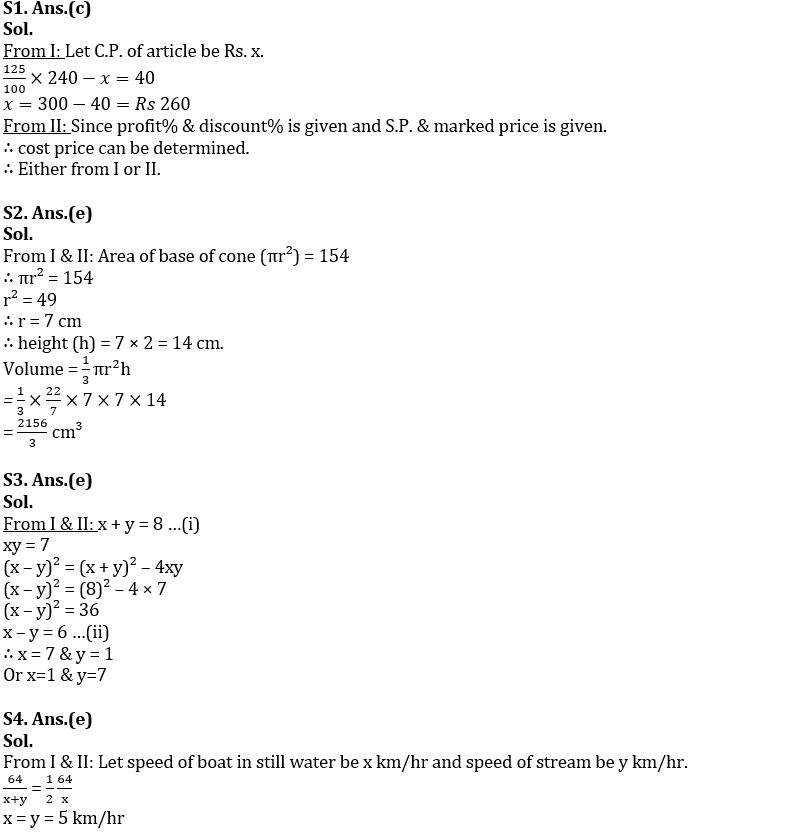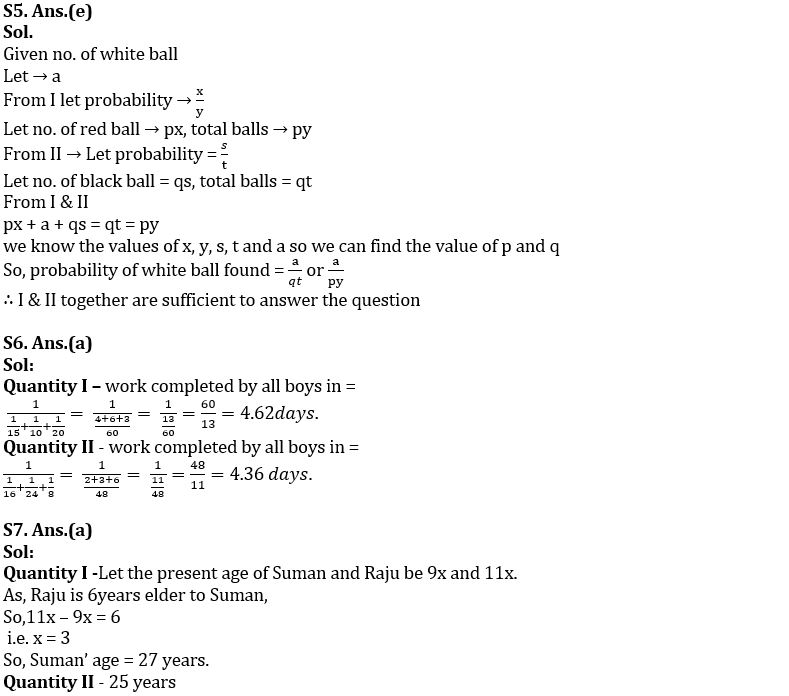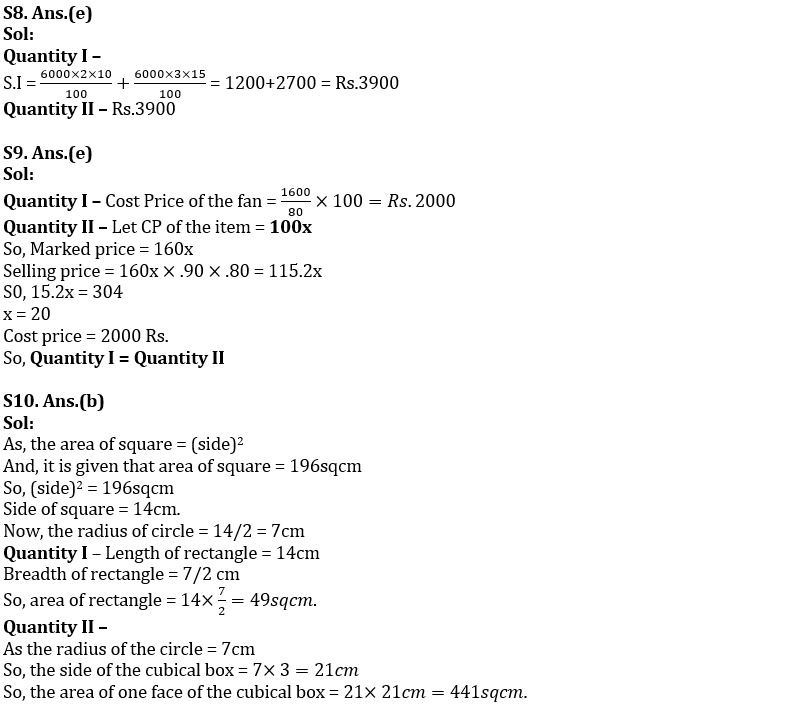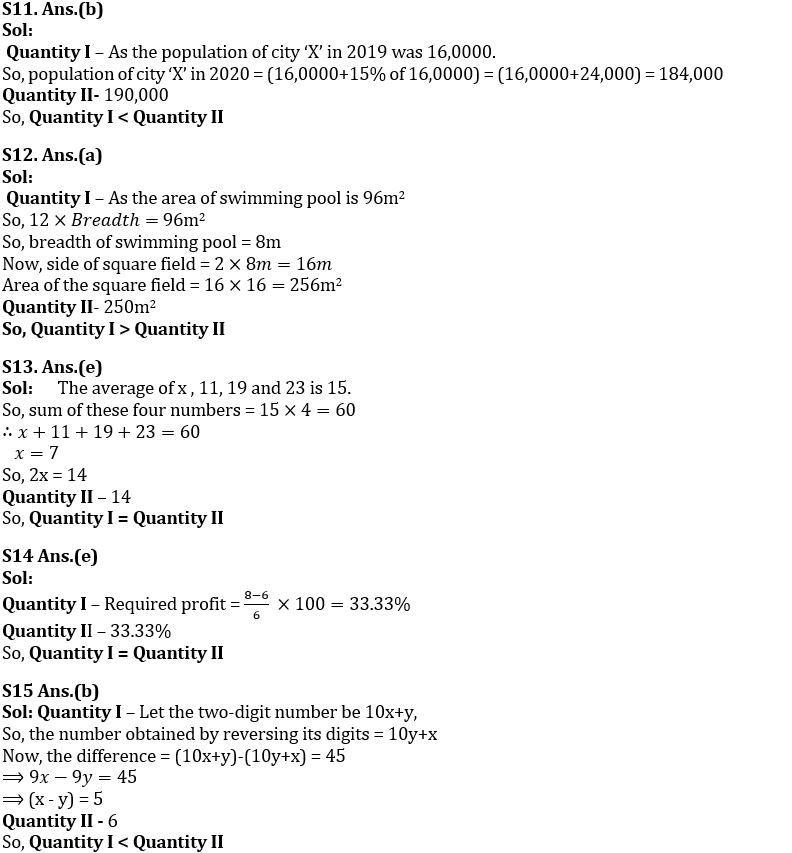## FAQs

### When will the SIDBI Grade A Exam 2023 be held?

The SIDBI Grade A Exam 2023 will be held on 28th January 2023.

#### Congratulations!